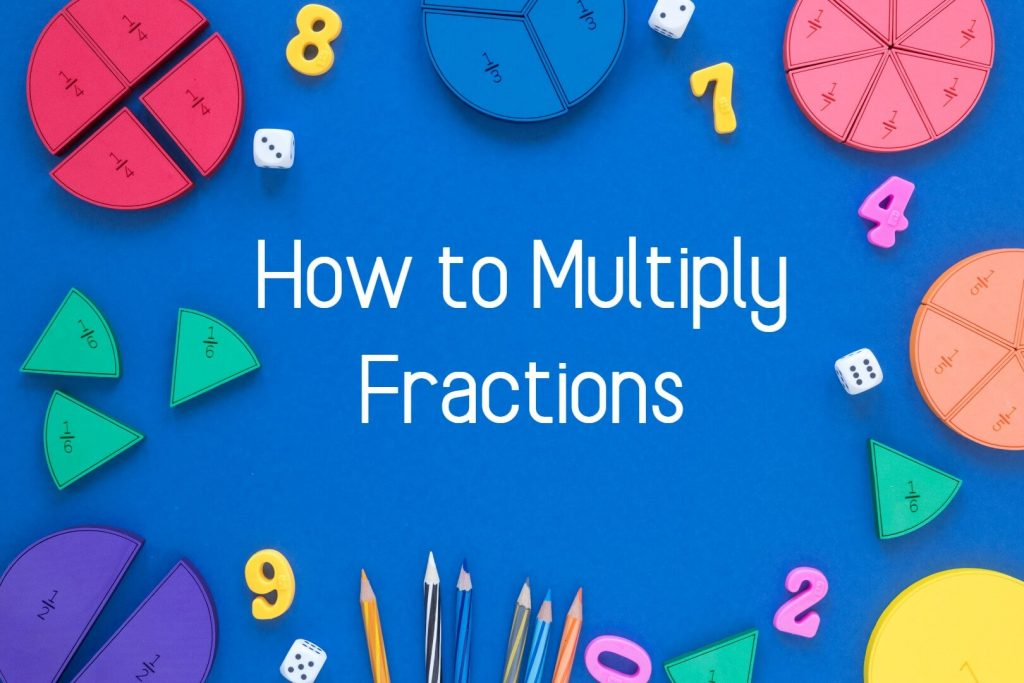# How to Multiply Fractions in 3 Steps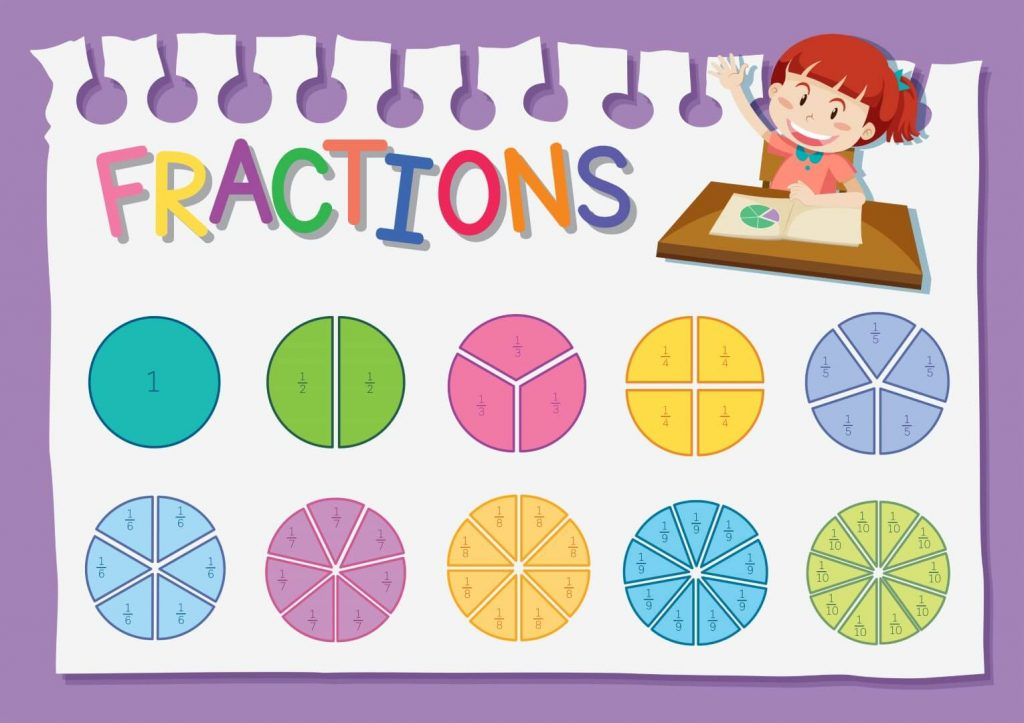## How to Multiply Fractions

Multiply fractions can discuss under three main categories.

1. Multiplication of proper fractions
2. Multiplication of improper fractions
3. Multiplication of a mixed numbers

## Multiplication of Proper Fractions

A proper fraction is a fraction that is less than one, with the numerator less than the denominator.

Example :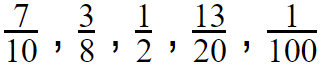### To multiply proper fractions you have to follow only three simple steps

Step 1 – Multiply the numerators
Step 2 – Multiply the denominators
Step 3 – Simply the fraction to it’s the simplest form (You have to follow this step, only when we have common factors for the numerator and denominator. Otherwise keep it as it is. )

Now let’s discuss a few simple examples

Example 01 :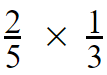Now let’s solve this one by following the above three simple steps.

Step 01 and Step 02  ( if it is difficult for you to multiply you can use a multiplication table )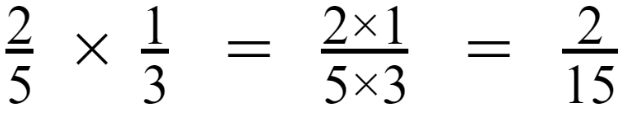Step 03 –

By following step 01 and step 02 we got our answer as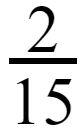In step 03, we have to simplify the fraction. But in the above answer, we can see that there are no common factors (a number which divides both 2 and 15).

So the final answer will be,Example 02 :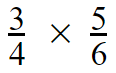Step 01 and Step 02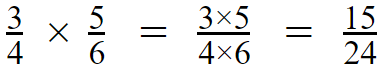Now we got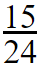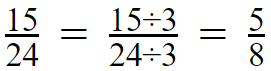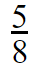Example 03 :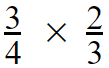Step 01 and Step 02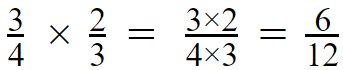Step 03

For this numerator and denominator, we have several common factors such as 2,3 and 6.

out of those factors you have to select the greatest common factor (and also called as the Highest Common Factor – HCF)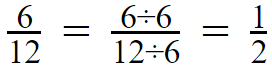So the final answer will be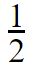Think that you cannot find the greatest common factor,
which is 6 in the above example. Don’t get confused. You can continue to simplify the fraction with any common factor that you remember.

Let’s think that you could only find 2 as a common factor. Just continue with 2.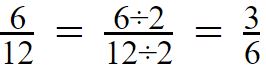In our new fraction which is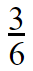again, we can see that 3 is a common factor.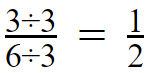Now you will get the same answer. These are equivalent fractions.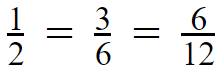## Multiplication of Improper Fractions

An Improper Fraction is a fraction in which the numerator is greater than the denominator

Example: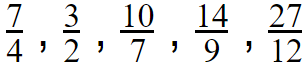### To multiply improper fractions you have to follow only three simple steps

Step 1 – Multiply the numerators
Step 2 – Multiply the denominators
Step 3 – Convert the improper fraction into a mixed number

Now let’s discuss a few simple examples

Example 1 :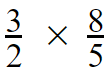Step 01 and Step 02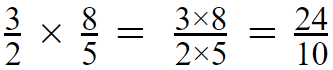Step 03

We cannot keep the answer as an improper fraction, so we have to convert it into a mixed number. There are several methods to do the conversion.

Let me explain an easy method for you.

In this method, You have to use the long division. Divide the numerator by denominator.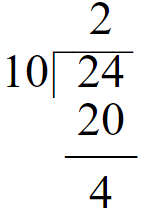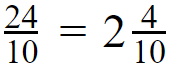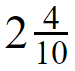Example 02 :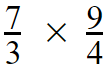Step 01 and Step 02Step 03

Divide 63 by 12, Then the quotient is 5 and the remainder is 3.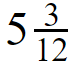## Multiplication of a Mixed Numbers

A number which is the sum of a whole number and a proper fraction written in this manner defined as Mixed Numbers

Examples :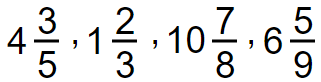### To multiply mixed numbers you have to follow four simple steps

Step 1 – Convert the mixed number into improper fraction
Step 2 – Multiply the numerators
Step 3 – Multiply the denominators
Step 4 – Express the answer as a mixed number

Example 01 :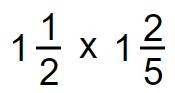Step 01

Write each mixed number as an improper fraction.Step 02 and Step 03

Multiply numerator by numerator and denominator by denominator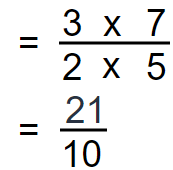Step 04

Convert the improper fraction to mixed number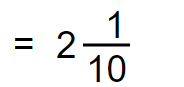Example 02 :

You can remove the common factors as follows.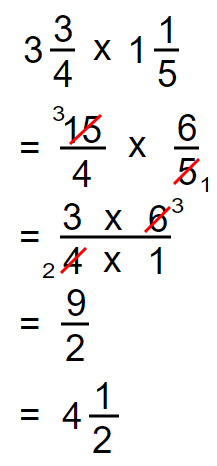Example 03 :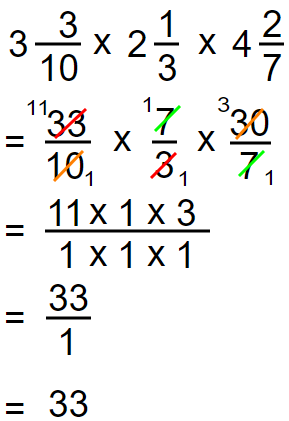Now you have knowledge of how to multiply fractions. Also you have to practice,

• how to multiply mixed fractions with different denominators
• how to multiply fractions by fractions
• how to divide and multiply fractions
• how to multiply two fractions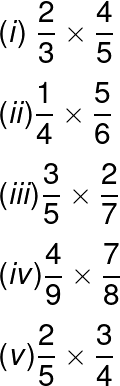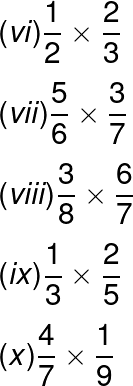1.